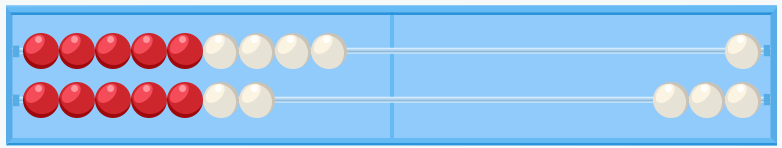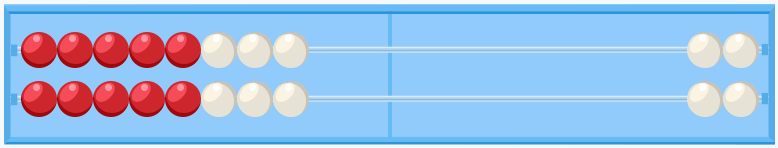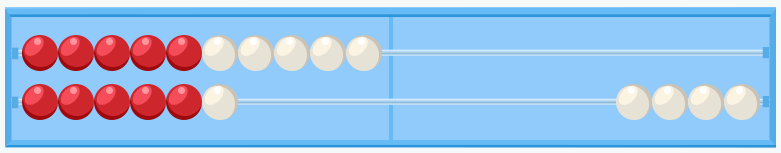Smartick is an advanced online program that teaches kids math and coding in only 15 min. a day

Apr02

# Learn to Add Using the Rekenrek

In today’s post we’re going to learn to add using the Rekenrek abacus to help us.

We saw in a previous post that the rekenrek is a flexible learning material because it can be adapted to different calculation strategies.

Today we’re going to talk in detail about how to use the rekenrek to help demonstrate some of the most popular addition strategies. Once you’ve seen them all, you can decide which one is the best to use for the problem you want to solve.

We’re going to learn to add 9 + 7 using the two most well-known strategies.

#### Doubles with the rekenrek

To solve the addition 9 + 7, first we need to represent both numbers on the rekenrek: we put the 9 on the top row and the 7 on the bottom row, placing all the balls on the left.Now we can calculate the sum of both numbers by counting the total number of balls on the left of the rekenrek. There are 16 in total.

However, if you want to start using a strategy that allows you to dispense with counting and start using subitization, you can remove a ball from the top row and add one to the bottom row. You’ll have the same number represented, but in the following manner:It’s very easy to recognize the number represented on both rows with 5 red balls and 3 white ones. Have you got it? Of course! It’s 8 (5 and 3).

Therefore, you’ll recognize the total number represented as the double of 8, since it’s represented twice – on the top row and the bottom. This number, as I’m sure you know, is 16.

So, we’ve managed to calculate 9 + 7 as the double of 8, using the “doubles” strategy:

9 + 7 = (8 + 1) + 7= 8 + (1 + 7) = 8 + 8 = 16

#### Counting up to a ten with the rekenrek

Now we’re going to solve the same addition, 9 + 7, using a different strategy. You might find it more natural, since 9 is very close to a ten.

As before, we represent both summands on the rekenrek:Now, since we only need one more ball to make a ten on the top row, we remove a ball from the bottom row and add one to the top row. Now we have a ten on the top row, and the balls are arranged like this:Since we’ve filled in the top row, we’ve represented the 10. On the other hand, it’s also easy to see how many balls there are on the bottom row, since there are 5 red balls and 1 white one, making 6 (5 and 1).

So, as you can see, we’ve represented 16.

We’ve managed to calculate 9 + 7 as 10 and 6, using the “counting up to the ten” strategy:

9 + 7 = 9 + (1 + 6) = (9 + 1) + 6 = 10 + 6 = 16

What do you think of the rekenrek as an addition aid? Which strategy did you think was easier? Leave us a comment and let us know!

The rekenrek allows us to vizualize the numbers “at a glance”, internalizing the subitization. In other words, it helps us recognize the visual representation of each number quickly.

If you want to learn to add with the rekenrek and practice other math exercises, log in to Smartick and try it free.